# Histogram

(diff) ← Older revision | Latest revision (diff) | Newer revision → (diff)

A method for representing experimental data. A histogram is constructed as follows. The entire range of the observed valuesof some random variableis subdivided intogrouping intervals (which are usually all of equal length) by points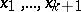; the number of observations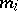per interval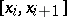and the frequencyare computed. The pointsare marked on the abscissa, and the segments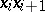(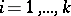) are taken as the bases of rectangles with heights. If the intervalshave equal lengths, the altitudes of the rectangles are taken as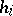or as. Thus, let the measurements of trunks of 1000 firs give the following results:'

<tbody> </tbody>
 diameter in cm. 22–27 27–32 32–37 37–42 42–52 number of trunks 100 130 500 170 100

The histogram for this example is shown in the figure. diameter in cm. number of trunksFigure: h047450a

The histogram can be considered as a technique of density estimation (cf. also Density of a probability distribution), and there is much literature on its properties as a statistical estimator of an unknown probability density asand the grouping intervals are made smaller (grouping intervals of lengths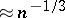seem optimal).
 [a1] D. Freedman, P. Diaconis, "On the histogram as a density estimator: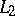theory" Z. Wahrsch. Verw. Geb. , 57 (1981) pp. 453–476